Acid Base Titrations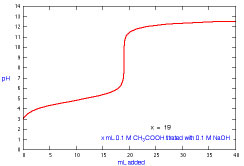How does a titration curve change when the mL of acid is changed?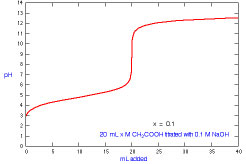How does a titration curve change when the molarity of acid is changed?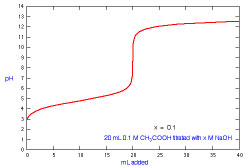How does a titration curve change when the molarity of base is changed?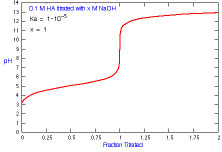How does a titration curve change when the concentration of titrant is changed?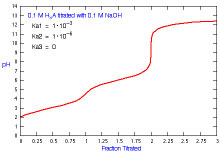How does a titration curve change when there is more than one Ka?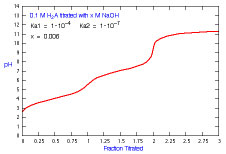How does a titration curve change when the concentration of titrant is changed and there is more than one Ka?What species are present during the titration of acetic acid?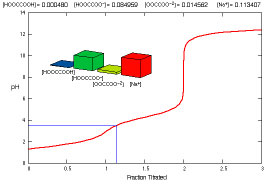What species are present during the titration of oxalic acid?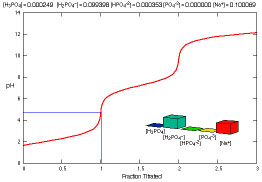What species are present during the titration of phosphoric acid?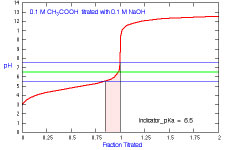What is the appropriate indicator to use for a titration of acetic acid?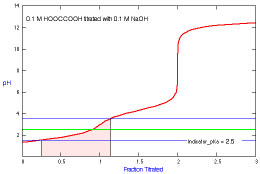What is the appropriate indicator to use for a titration of oxalic acid?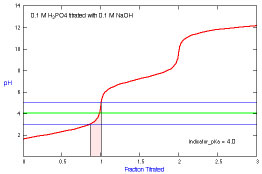What is the appropriate indicator to use for a titration of phosphoric acid?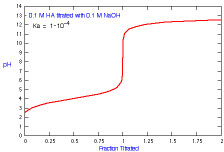How does a titration curve change when Ka is changed?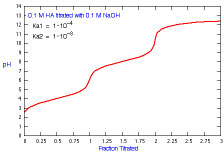How does a titration curve change when Ka2 is changed?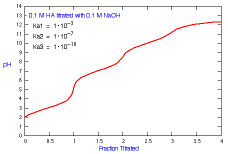How does a titration curve change when Ka3 is changed?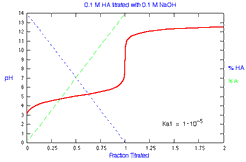What is the distribution of species during titration of a monoprotic acid? How does the distribution change with Ka?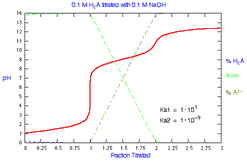What is the distribution of species during titration of a diprotic acid? How does the distribution change with Ka1?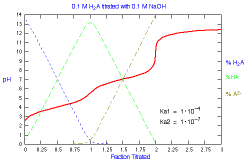What is the distribution of species during titration of a diprotic acid? How does the distribution change with Ka2?